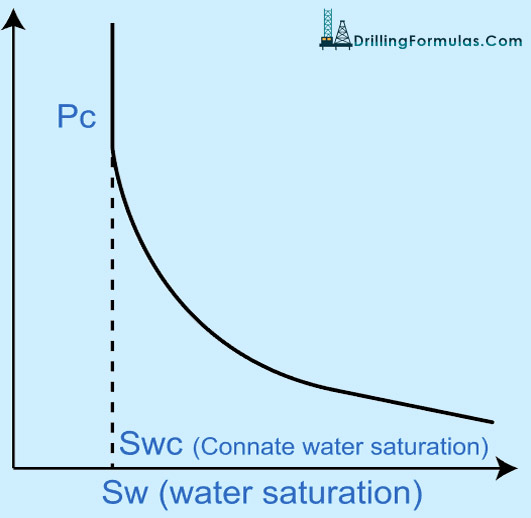# Capillary Pressure

Capillary pressure is a force due to differentials between fluid densities in a rock that can force pull hydrocarbon through the pores of a rock so a transition zone between fluids occurs.

Let’s make it simple. If we put a small tube in water overlaid by oil, water will rise up into the tube due to capillary pressure (Pc). For this situation, the capillary pressure (Pc) is the difference in pressure across the curved interface between the fluids (oil and water) shown in Figure 1.Figure 1 – Capillary Diagram

Pc in Figure 1 can be expressed as

Pc = 2 × σwo × Cos (Ɵ) ÷ r   equation 1

Where;

σwo is interfacial tension between fluids.

Ɵ is a contact angle.

r is a radius of tube.

With the simple diagram in Figure 1, it demonstrates that smaller pore size (small r) will have a higher capillary pressure than bigger pore sized rock.

When fluid is in a static condition, then gravitational force equates to capillary force. Then, the capillary pressure can be defined as the difference between fluid densities.

Pc = (ρw − ρo) ×g ×h —- equation 2

Where;

ρw = water density

ρo = oil density

g = gravitational acceleration

h = water column height

Distribution of fluid in a reservoir rock depends on wettability phase saturation. It means than less wetting phase saturation will have a higher capillary pressure because it occupies small spaces of rock. Figure 2 shows a relationship between Pc and wetting phase saturation.Figure 2 – Capillary Pressure and Sw

A reservoir with oil water contact will have a transition zone from free water zone (100% Sw) to connate water saturation, Swc, in an oil reservoir due to capillary pressure.Figure 3 – Transition Zone Diagram

Figure 3 demonstrates a transition zone diagram and height (h) of a transition zone above a free water level, and it can be calculated by the following equation.

h = (144 × Pc) ÷ (ρw − ρo)

h = transition zone height, ft

Pc = capillary pressure, psi

ρw = water density, lb/cu-ft

ρo = oil density, lb/cu-ft

This concept is very important because in some reservoirs that have oil water contact, there is no clear cut difference between oil and water because the capillary pressure creates a transition zone. The bigger transition zone may cut down the oil reserve in reservoir dramatically in some cases.

Example:  A core sample is tested to determine the capillary pressure. The capillary pressure at reservoir condition is 20 psi and the difference in density of servitor oil and water is 17.0 lb/ct. The rock has Sw of 30%. What is a transition zone height at Sw 30%?

h = (144 × Pc) ÷ (ρw − ρo)

h = (144 × 20) ÷ (17)

h = 169.4 ft.

As you can see from this simple calculation, the transition zone is almost 170 ft. If you have 600 ft of sand, 28% of the reservoir is in a transition zone.

References

Abhijit Y. Dandekar, 2013. Petroleum Reservoir Rock and Fluid Properties, Second Edition. 2 Edition. CRC Press.

L.P. Dake, 1983. Fundamentals of Reservoir Engineering, Volume 8 (Developments in Petroleum Science). New impression Edition. Elsevier Science.

Tarek Ahmed PhD PE, 2011. Advanced Reservoir Management and Engineering, Second Edition. 2 Edition. Gulf Professional Publishing.

Share the joy